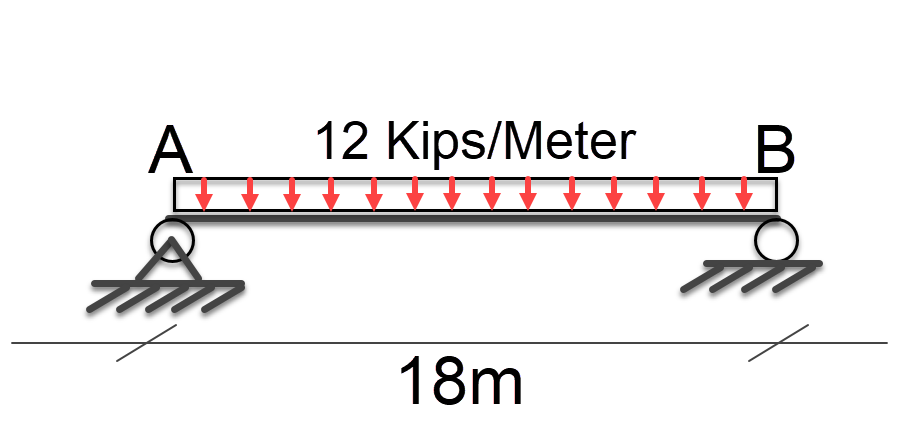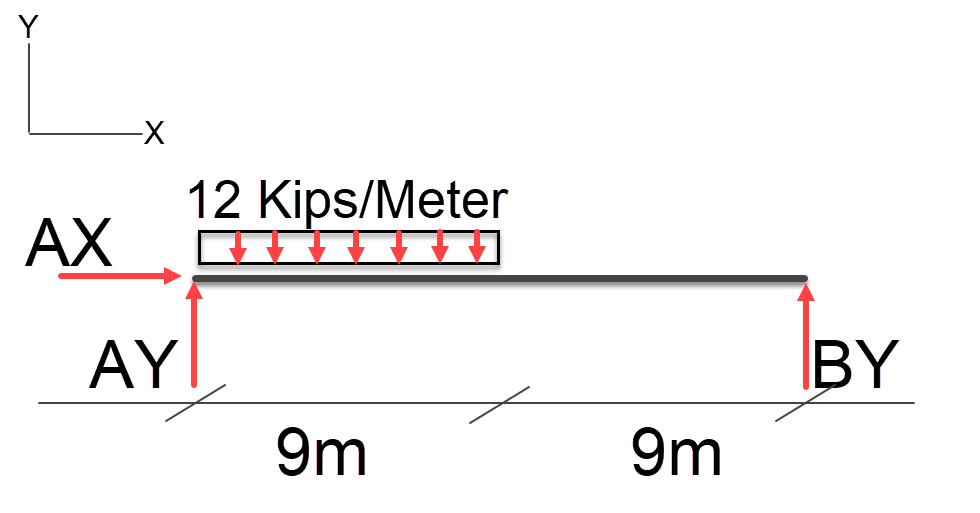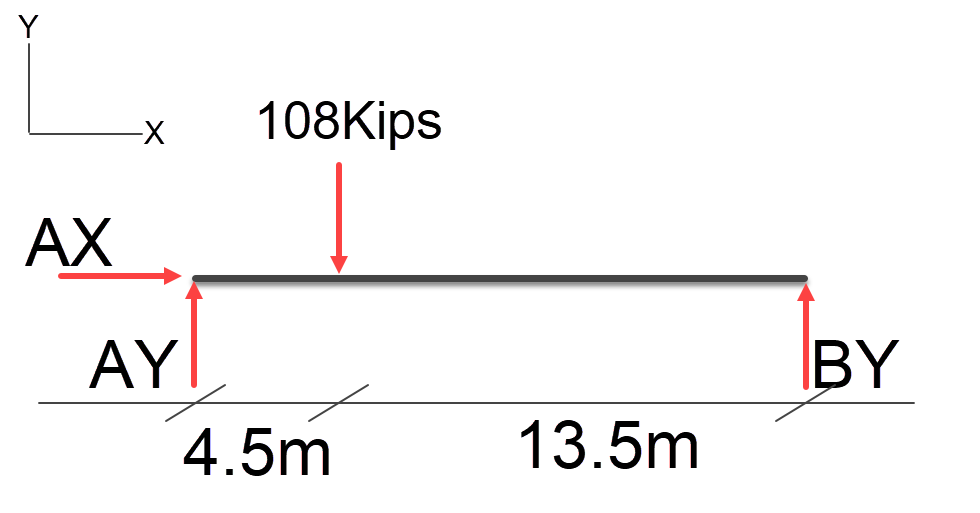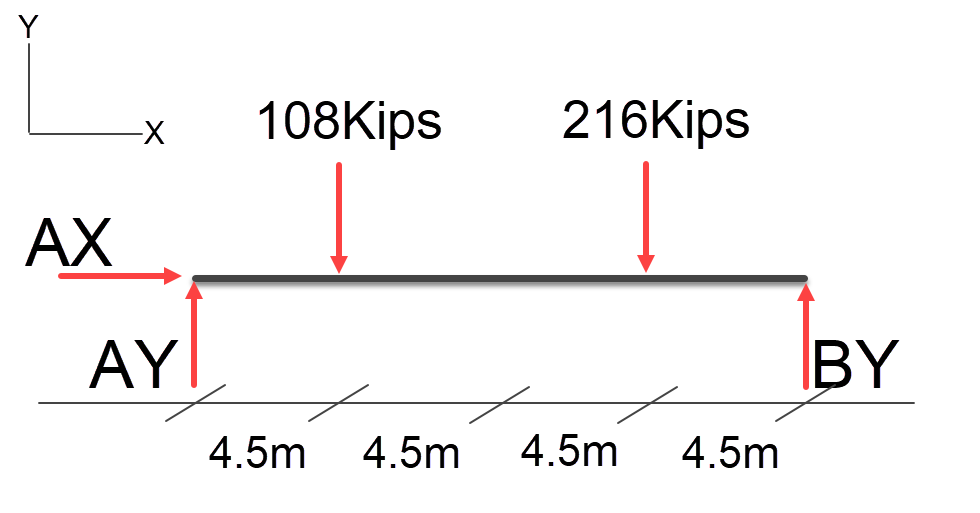# Resolving Distributed Loads on a Beam

Table of Contents

### Resolving Distributed Loads on a Beam

In a previous post we looked at resolving point loads on a beam in this post we will look at distributed loads.

In this example we will see how to resolve the resultant loads at A and B due to a 12Kips/Meter distributed load.

Tonnes in Kips = 2.2046Load Diagram

### Free Body Diagram

First we will create a free body diagram, to represent our problem.Free Body Diagram

### Converting a Uniform Distributed Load to a Point Load

It’s important to note that the AX load is 0kips in this case since no loads have any horizontal component.

In the case of a distributed load, we can convert the distributed load to a point load this is achieved by multiplying distributed load by the distance it’s applied across. Then positioning the point load at the midpoint of the uniform distributed load (as long as its truly uniform).

In our example we have an 18meter span with 12 kips / meter = 18 * 12 = 216kip point load at the mid point of the span.Free Body Diagram

Since its at the mid point of the span we can simply divide this by 2 to find the resultant loads at AY and BY = 108Kips.

### Offset Uniform Distributed Load

In this case the distributed load is offset to one side lets look at how we resolve this.Offset UDL Free Body Diagram

Using the same logic previously applied to convert a uniform distributed load, we can do the same for this example, first we multiple the uniform distributed load by its span 12kips * 9 = 108kip point load at the mid point of the distributed load this is shown below;Offset UDF as a Point Load Free Body Diagram

We can now resolve the resultant forces at AY and BY using the point load method.

1 / ( 4.5 + 13.5 ) = 0.0555555 \\
0.0555555 * 4.5 = 0.25 \\
108kips * 0.2 = 27kips \\

And we know that BY = Total Point Load – AY, so BY = 81Kips.

We can also do the calculation by;

Load * Span / TotalSpan \\
AY = (108 * 4.5 ) / 18 \\
AY = 486 / 18 \\
AY = 27 \\
BY = (108 * 13.5 ) / 18 \\
BY = 1,458 / 18 \\
BY = 81 \\
Load = 27 +  81 = 108


### Multiple Offset Loads

In this case we have two uniform distributed loads lets see how we handle this;Multiple Uniform Distributed Loads Free Body Diagram

Just as before we convert the uniform distributed loads to point loads;Multiple Uniform Distributed Loads Converted to Point Loads Free Body Diagram

Now we can do the calculations, lets start of with AY;

Load * Span / TotalSpan \\
AY1 = (108 * 4.5 ) / 18 \\
AY1 = 486 / 18 \\
AY1 = 27 \\
AY2 = (216 * 13.5 ) / 18 \\
AY2 = 2916 / 18 \\
AY2 = 162 \\
AY1 + AY2 = 189kips


Next BY;

Load * Span / TotalSpan \\
BY1 = (108 * 13.5 ) / 18 \\
BY1 = 1458 / 18 \\
BY1 = 81 \\
BY2 = (216 * 4.5 ) / 18 \\
BY2 = 972 / 18 \\
BY2 = 54 \\
BY1 + BY2 = 135kips

And as a final check 189 + 135 = 324kips

And as they say that is that, next post will cover Non-Uniform Distributed Loads.